# Square Root In Excel (SQRT)Article byExcelMojo Team## What Is Square Root Formula In Excel (SQRT)?

The Square Root in Excel (SQRT) is an arithmetic function used to find the square root of a given number or an integer. The Excel Square Root is an inbuilt “Math and Trig” function, so we can insert the formula from the “Function Library” or enter it directly in the worksheet.

For example, the image depicts the set of values, and we will calculate Square Root in Excel using the SQRT formula.

Select cell B2, enter the formula =SQRT(A2), and press the “Enter” key.

The result in cell B2 is “100”, as shown above.

###### Key Takeaways
• The Square Root in Excel helps us find the square root of any given numeric value. The value can be a negative or a positive integer.
• If we get an error as a value is negative, then we can use the ABS function to get the precise result.
• We can use the function along with other functions such as ABS, ROUND, ROUNDUP, and ROUNDDOWN.
• The SQRT function is similar to the POWER function, which works like an exponent.
• If we enter a numeric value, we can also get the square root of that number using the power query method.

### Negative Square

The Square root of numbers can be negative as well as positive.

For example,

• -5 * -5

= 5 * 5 (If we multiply the negative sign by the negative sign, it becomes a positive sign, and the positive number is left.)

=25

• A square of a positive number is also a positive number.

= 5 * 5

=25

Therefore, the Square Root of 25 can either be 5 or -5.

### Syntax Of Square Root (SQRT) In Excel

The syntax of the Square Root(SQRT) formula is,

The mandatory argument of the Square Root formula is,

• number: It is the numeric value we choose to find the square root. The number should be a positive number or one that results in a positive number.

### How To Calculate Square Root (SQRT) In Excel?

The Square Root function is used in 2 ways, namely,

1. Access from the Excel ribbon.
2. Enter in the worksheet manually.

#### Method #1 – Access from the Excel ribbon

First, choose an empty cell → select the “Formulas” tab → go to the “Function Library” group → click the “Math & Trig” option drop-down → select the “SQRT” function, as shown below.

The “Function Arguments” window opens. Enter the arguments in the “Number” field, and click “OK”, as shown below.

#### Method #2 – Enter in the worksheet manually

1. Choose an empty cell for the output.
2. Type =SQRT( in the cell. [Alternatively, type =S or =SQ, and select the SQRT function from the suggestions given by Excel]
3. Enter the arguments as cell values or cell references in excel.
4. Close the brackets, and press the “Enter” key to execute the formula.

The following example depicts the set of values, and we will calculate the Square Root using the SQRT Excel formula.

In the table, the data is,

• Column A contains the Value.
• Column B contains the Output.

The steps to evaluate the values to find the Square Root in excel are as follows:

1. Select cell B2, and enter the formula =SQRT(A2).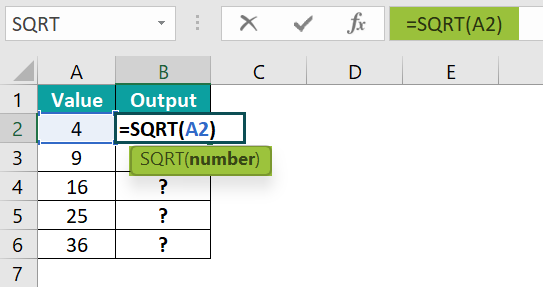2. Press the “Enter” key. The result is “2”, as shown below.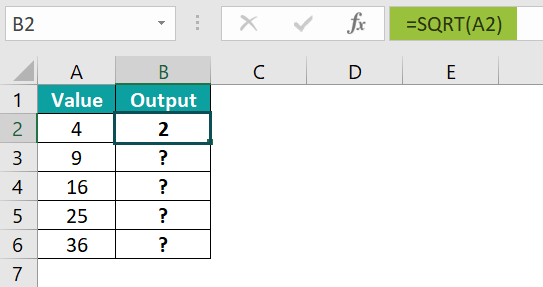3. Drag the formula from cell B2 to B6 using the fill handle. The output is shown below.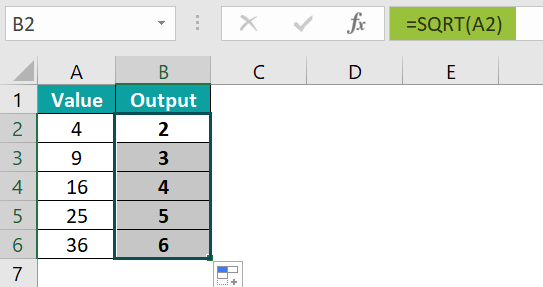### Examples

We will consider some advanced scenarios using the Square Root in Excel examples.

#### Example #1

The following example depicts the set of values, and we will calculate Square Root using the SQRT Excel formula.

In the table, the data is,

• Column A contains the Value.
• Column B contains the Output.

The steps to evaluate the values to find the Square Root are as follows:

• Step 1: Select cell B2, enter the formula =SQRT(A2), and press the “Enter” key. The result is “5”, as shown below.
• Step 2: Drag the formula from cell B2 to B6 using the fill handle. The output is shown below.

#### Example #2

The following example depicts the set of negative values, and we will calculate Square Root using the SQRT Excel formula with the ABS Excel formula.

In the table, the data is reflected below: –

• Column A contains the Value.
• Column B contains the Output.

The steps to evaluate the values to find the Square Root are as follows:

• Step 1: Select cell B2, enter the formula =SQRT(ABS(A2)), and press the “Enter” key. The result is “2”, as shown below.
• Step 2: Drag the formula from cell B2 to B6 using the excel fill handle. The output is shown below.

#### Example #3

The following example depicts the set of values, and we will calculate Square Root using the Excel formula.

In the table, the data is,

• Column A contains the Value.
• Column B contains the Output.

The steps to evaluate the values to find the Square Root are as follows:

• Step 1: Select cell B2, and enter the formula = A2 ^ (1/2).
• Step 2: Press the “Enter” key. The result is “3.1622777”, as shown below.
• Step 3: Drag the formula from cell B2 to B5 using the fill handle. The output is shown below.

### Important Things To Note

• The #VALUE! error occurs when we enter anything other than the numerical value because the SQRT function accepts only numbers.
• The #NUM! error occurs when we enter negative numbers because the SQRT function accepts only positive numbers.
• The shortcut to insert the square root symbol is to hold the “ALT” key and type 251 from the number keypad of the keyboard.

1. How do I do Square Root in Excel?

The following example depicts the set of values, and we will calculate Square Root in Excel using the SQRT Excel formula.

In the table, the data is,
Column A contains the Value.
Column B contains the Output.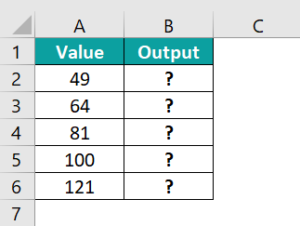The steps to evaluate the values to find the Square Root in Excel are as follows:

• Step 1: Select cell B2, enter the formula =SQRT(ABS(A2)), and press the “Enter” key. The result is “7”, as shown below.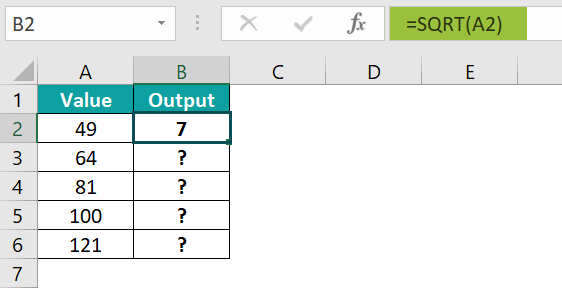• Step 2: Drag the formula from cell B2 to B6 using the fill handle. The output is shown below.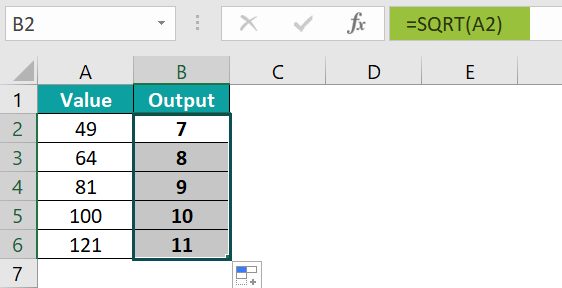2. How to Calculate the Square Root of a Number Using the POWER Function?

The Square Root of a Number using the POWER Function illustrates the number of times a number is multiplied by itself.

The syntax of the POWER function is =POWER(number,power)

The following example depicts the set of values, and we will calculate Square Root in Excel using the POWER Excel formula.

In the table, the data is,
Column A contains the Value.
Column B contains the Output.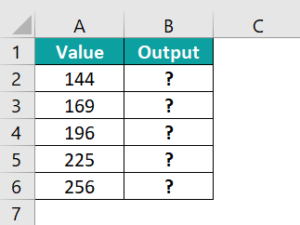The steps to evaluate the values by calculating Square Root in Excel using the POWER function are as follows:

• Step 1: Select cell B2, enter the formula =POWER(A2,1/2), and press the “Enter” key. The result is “12”, as shown below.

[Note: The cell that contains a number, i.e., “A2”, and the cell that contains power, i.e., “1/2”.]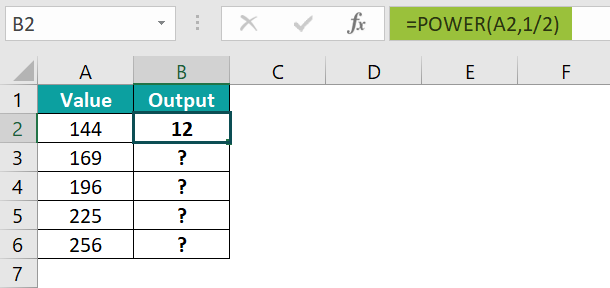• Step 2: Drag the formula from cell B2 to B6 using the fill handle. The output is shown below.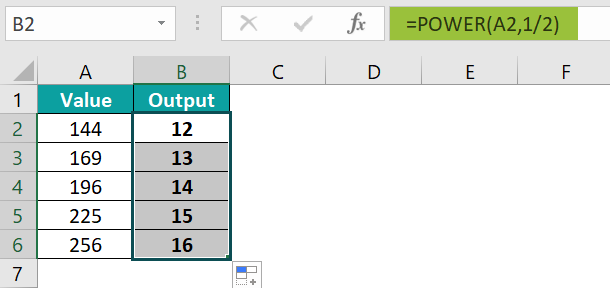3. What is the shortcut of the Square Root in Excel (SQRT)?

The shortcut of the Square Root in Excel (SQRT) is as follows: